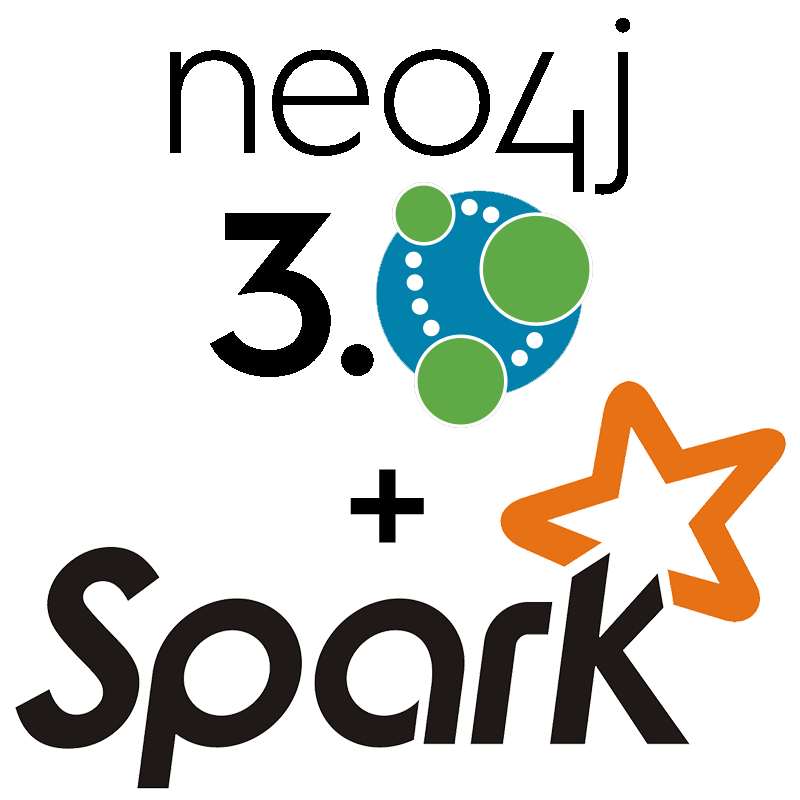# Introducing the Neo4j 3.0 Apache Spark ConnectorWe proudly want to participate in this week’s flurry of announcements around Apache Spark.

While we’re cooperating with Databricks in other areas like the implementation of openCypher on Spark and as an industry-partner of AMPLab, today I want to focus on the Neo4j Spark Connector.

### Enabled by Neo4j 3.0

One of the important features of Neo4j 3.0 is Bolt, the new binary protocol with accompanying official drivers for Java, JavaScript, .NET and Python. That caused me to give implementing a connector to Apache Spark a try, and also to see how fast I can transfer data from Neo4j to Spark and back again.

The implementation was really straightforward. All the interaction with Neo4j is as simple as sending parameterized Cypher statements to the graph database to read, create and update nodes and relationships.

### Features of the Spark Connector

So I started with implementing a Resilient Distributed Dataset (RDD) and then added the other Spark features, including GraphFrames, so that the connector now supports:

• RDD
• DataFrame
• GraphX
• GraphFrames

You can find more detailed information about it’s usage here; this is only a quick overview on how to get started.

### Quickstart

For a simple dataset of connected people, run the following two Cypher statements that create 1M people (with `:Person` labels and `id`, `name` and `age` attributes) and 1M `:KNOWS` relationships, all in about a minute.

```UNWIND range(1, 1000000) AS x
CREATE (:Person {id: x, name: 'name' + x, age: x % 100}))
```

```UNWIND range(1, 1000000) AS x
MATCH (n)
WHERE id(n) = x
MATCH (m)
WHERE id(m) = toInt(rand() * 1000000)
CREATE (n)-[:KNOWS]->(m)
```

### Spark Shell

Now we can start both spark-shell with our connector and GraphFrames as packages.

```\$SPARK_HOME/bin/spark-shell \
--packages neo4j-contrib:neo4j-spark-connector:1.0.0-RC1,\
graphframes:graphframes:0.1.0-spark1.6
```

And to start using it, we only do a quick RDD and GraphX demo and then look at GraphFrames.

### RDD Demo

```import org.neo4j.spark._

// statement to fetch nodes with id less than given value
val query = "cypher runtime=compiled MATCH (n) where id(n) < {maxId} return id(n)"
val params = Seq("maxId" -> 100000)

Neo4jRowRDD(sc, query, params).count
// res0: Long = 100000
```

### GraphX Demo

```import org.neo4j.spark._

val g = Neo4jGraph.loadGraph(sc, label1="Person", relTypes=Seq("KNOWS"),  label2="Person")
// g: org.apache.spark.graphx.Graph[Any,Int] = org.apache.spark.graphx.impl.GraphImpl@574985d

// What's the size of the graph?
g.vertices.count          // res0: Long = 999937
g.edges.count             // res1: Long = 999906

// let's run PageRank on this graph
import org.apache.spark.graphx._
import org.apache.spark.graphx.lib._

val g2 = PageRank.run(g, numIter = 5)

val v = g2.vertices.take(5)
// v: Array[(org.apache.spark.graphx.VertexId, Double)] =
//    Array((185012,0.15), (612052,1.0153), (354796,0.15), (182316,0.15), (199516,0.385))

// save the PageRank data back to Neo4j, property-names are optional
Neo4jGraph.saveGraph(sc, g2, nodeProp = "rank", relProp = null)
// res2: (Long, Long) = (999937,0)
```

### GraphFrames Demo

```import org.neo4j.spark._

val labelPropertyPair =   ("Person" -> "name")
val relTypePropertyPair = ("KNOWS" -> null)

val gdf = Neo4jGraphFrame(sqlContext, labelPropertyPair, relTypePropertyPair, labelPropertyPair)
// gdf: org.graphframes.GraphFrame = GraphFrame(v:[id: bigint, prop: string],
//                                              e:[src: bigint, dst: bigint, prop: string])

gdf.edges.count           // res2: Long = 999999

// pattern matching
val results = gdf.find("(A)-[]->(B)").select("A","B").take(3)
// results: Array[org.apache.spark.sql.Row] = Array([[159148,name159149],[31,name32]],
//               [[461182,name461183],[631,name632]], [[296686,name296687],[1031,name1032]])
```